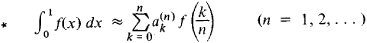# Cotes Integration Formulas

The following article is from The Great Soviet Encyclopedia (1979). It might be outdated or ideologically biased.

## Cotes Integration Formulas

formulas used for approximating definite integrals using the values of the integrand at a finite number of equally spaced points, that is, quadrature formulas with equally spaced nodes. The Cotes integration formulas have the formThe numbers ak(n) are called the Cotes coefficients and are determined under the condition that the formula (*) is exact whenever f( x) is a polynomial of degree not greater than n. The Cotes integration formulas were introduced by the British mathematician R. Cotes (1682–1716).